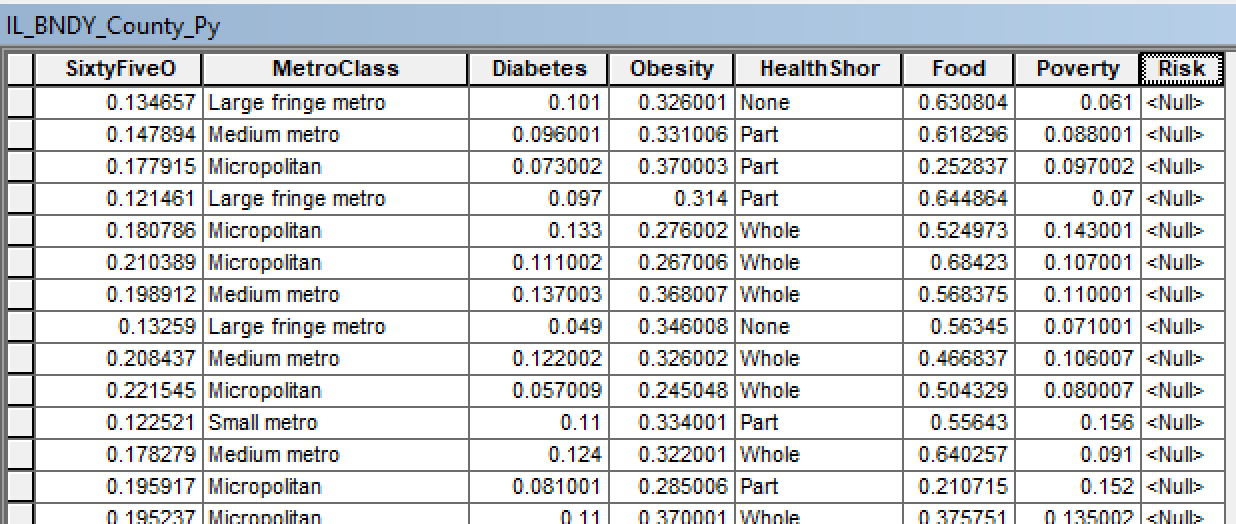# Help using ArcGIS Desktop Field Calculator and Python to define risk classes.

315
1
04-20-2020 02:06 PMby
New Contributor II

I am trying to use if, elif, else statements in Field Calculator to define risk areas (low, moderate, high).  I attached an image of part of the attribute table.  I will be using all 7 classes in my risk determination, but I am only listing a few in my example below to give an idea of what I need to do.

I need to say things like:

if SixtyFiveO is less than or equal to '0.0933' and if MetroClass equals 'Noncore' or 'Micropolitan' and if Diabetes is less than '0.05', etc. then the risk column value is 'low'.

elif SixtyFiveO is greater than or equal to '0.09331' and less than or equal to '0.01866' and if MetroClass equals 'Small Metro' or 'Medium Metro' etc. then the risk column value is 'moderate'.

else...then the risk column value is 'high'.

I am not entirely sure how to write this.  Any help is much appreciated!Tags (1)New Contributor III

There is an example of this coding on the 'Calculate Field Example' page in the help called 'Calculate fields using logic with python'.

Use a Code Block similar to this:

``````def calcRisk(sixtyFiveO, metroClass, diabetes):
if sixtyFiveO <= 0.0933 and metroClass in ('Noncore', 'Micropolitan') and  Diabetes < 0.05:
theRisk = 'low'
elif sixtyFiveO >= 0.09331 and sixtyFiveO <= 0.0.01866 and metroClass in ('Small Metro', 'Medium Metro'):
theRisk = 'moderate'
else:
theRisk = 'high'

return theRisk‍‍‍‍‍‍‍‍‍``````

With a call to the code block like:

```calcRisk(!SixtyFiveO!, !MetroClass!, !Diabetes!)
‍‍```

Mark.